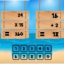Hlavní navigace

#OperationalMaths 1.0

OperationalMaths app is an exciting entertainment app. It is a fun and entertainment learning app for preschool children and toddlers including those with autism. It will learn simple mathematical equation solving into this app.

How to play?

> Player have one mathematical equation of addition operation and also have custom keyboard for type the answer of given equation so player can solve given equation and type the answer of that equation by custom keyboard into the 60 seconds once player done with answer at time player can tap one the “Check Answer” button for check the status of the answer.
> If player not Abel to solve equation in 60 seconds at time it will display another equation in to the screen for solve.
> Player get 5 points when successfully solve single addition equation.

2. Multiplication
> Player have one mathematical equation of multiplication operation and also have custom keyboard for type the answer of given equation so player can solve given equation and type the answer of that equation by custom keyboard into the 60 seconds once player done with answer at time player can tap one the “Check Answer” button for check the status of the answer.
> If player not Abel to solve equation in 60 seconds at time it will display another equation in to the screen for solve.
> Player get 5 points when successfully solve single multiplication equation.

Try it free for understanding mathematical equation solving with batter way of learning.

## Celkové hodnocení

Průměr hodnocení
3

Pro hodnocení programu se prosím nejprve

## Souhrnné informace o OperationalMaths

1.0

ne

29,97 MB

• Angličtina
• #### Staženo

0× celkem
0× tento měsíc

15. 5. 2020

## Něco jsme propásli?

Dejte nám vědět. Upozornit redakci Stahuj
Velice děkujeme za Vaše podněty